# Smart Pool: Alexa Controlled Pool Manager

Build your own high-quality water sensor to monitor your pool or spa, using the Amazon Alexa to check on the status of your pool.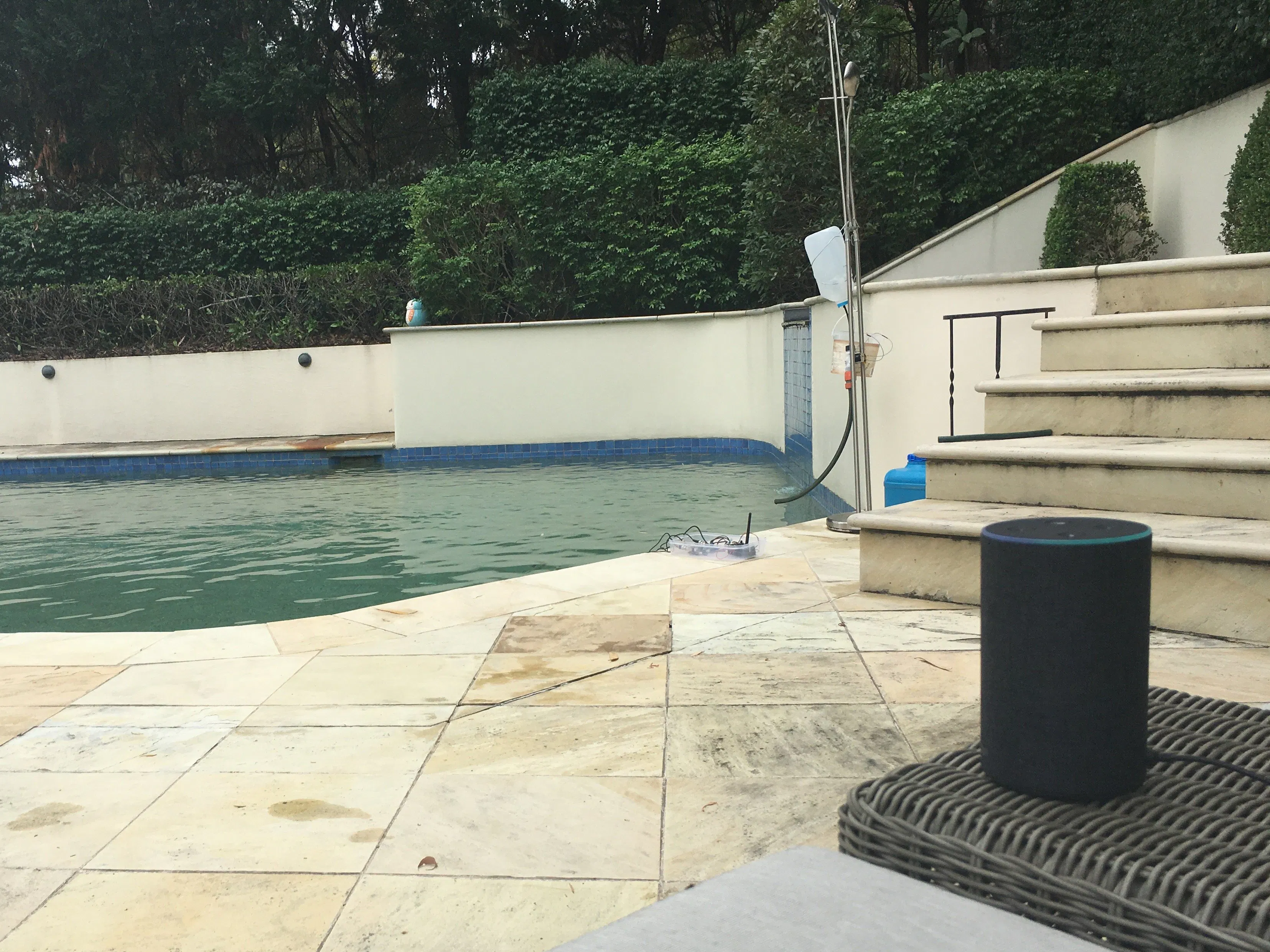## Things used in this project

### Hardware components

 Thinxtra xKit
×1Arduino UNO & Genuino UNO
×1
 Arduino Compatible Sensor Expansion Shield
×1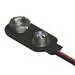9V Battery Clip
×2
 DFRobot Gravity: Analog ORP Sensor Meter For Arduino
×1
 DFRobot Gravity: Analog Electrical Conductivity Sensor / Meter For Arduino
×1
 pH Sensor Module + Electrode Probe for Arduino
×1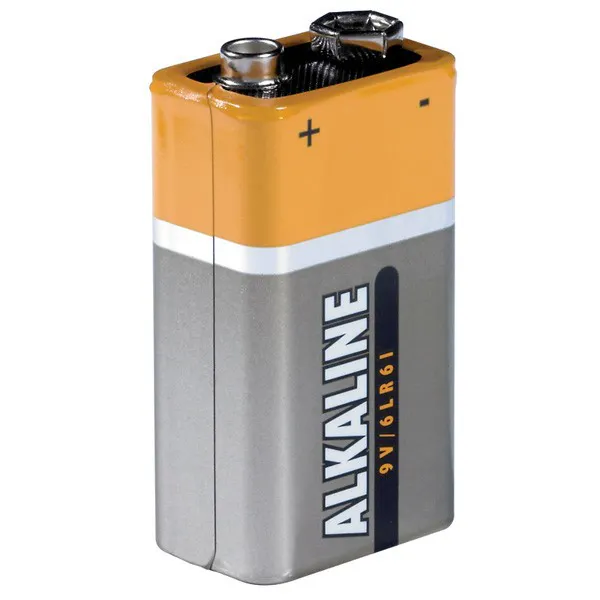9V battery (generic)
×2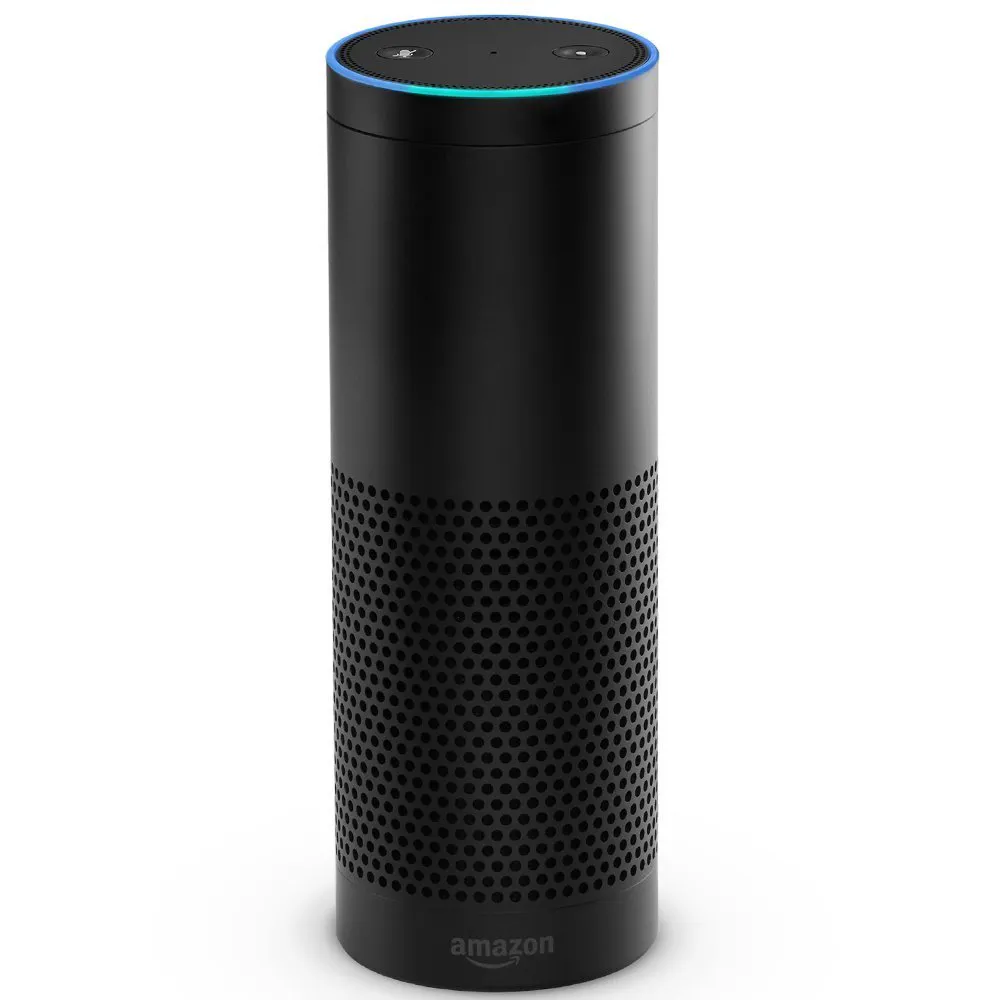Amazon Alexa Amazon Echo
×1
 Diode Rectifier
×1
 5V 1-Channel Relay Board Module
×1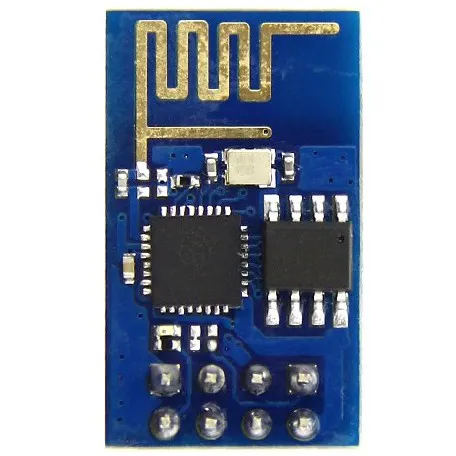Espressif ESP8266 ESP-01
×1
 12V Solenoid Valve - 3/4"
×1Jumper wires (generic)
×1

### Software apps and online servicesAmazon Web Services AWS LambdaAmazon Alexa Alexa Skills KitAmazon Web Services AWS DynamoDBArduino IDESigfoxAmazon Web Services AWS IoTAmazon Web Services AWS IAMIFTTT Maker serviceIFTTT Amazon Alexa service

## Code

### Combined_Sensors.ino

Arduino
```//Initilallising the libraries needed
#include <OneWire.h>
#include <Tsensors.h>
#include <Wire.h>
#include <Isigfox.h>
#include <WISOL.h>
#include <math.h>
#include <avr/wdt.h>

// Set up the protocol for Sigfox and the Sensors on board the xKit
Isigfox *Isigfox = new WISOL();
Tsensors *tSensors = new Tsensors();

// Defining the unions for the payload package
typedef union{
float number;
uint8_t bytes;
} FLOATUNION_t;

typedef union{
uint16_t number;
uint8_t bytes;
} UINT16_t;

typedef union{
int16_t number;
uint8_t bytes;
} INT16_t;

//pH Probe Setup
float calibration = 20.86; //change this value to calibrate
int sensorValue = 0;
unsigned long int avgValue;
float b;
int buf,temp;
int ph;
int ecValue;
int orp;

//EC Probe Setup
#define StartConvert 0

const byte numReadings = 20;     //the number of sample times
byte ECsensorPin = A3;  //EC Meter analog output,pin on analog 1
byte DS18B20_Pin = 5; //DS18B20 signal, pin on digital 2
unsigned int AnalogSampleInterval=25,printInterval=700,tempSampleInterval=850;  //analog sample interval;serial print interval;temperature sample interval
byte index = 0;                  // the index of the current reading
unsigned long AnalogValueTotal = 0;                  // the running total
unsigned int AnalogAverage = 0,averageVoltage=0;                // the average
unsigned long AnalogSampleTime,printTime,tempSampleTime;
float temperature,ECcurrent;

//Temperature chip i/o
OneWire ds(DS18B20_Pin);

//ORP Probe Setup
#define VOLTAGE 5.00    //system voltage
#define OFFSET 22        //OFFSET from calibration
#define LED 13

double orpValue;

#define ArrayLenth  40    //times of collection
#define orpPin 0          //Analog Pin ORP Module is connected
int orpArray[ArrayLenth];
int orpArrayIndex=0;

double avergearray(int* arr, int number){
int i;
int max,min;
double avg;
long amount=0;
if(number<=0){
printf("Error number for the array to avraging!/n");
return 0;
}
if(number<5){   //less than 5, calculated directly statistics
for(i=0;i<number;i++){
amount+=arr[i];
}
avg = amount/number;
return avg;
}else{
if(arr<arr){
min = arr;max=arr;
}
else{
min=arr;max=arr;
}
for(i=2;i<number;i++){
if(arr[i]<min){

amount+=min;        //arr<min
min=arr[i];
}else {
if(arr[i]>max){
amount+=max;    //arr>max
max=arr[i];
}else{
amount+=arr[i]; //min<=arr<=max
}
}//if
}//for
avg = (double)amount/(number-2);
}//if
return avg;
}

void setup() {
Serial.begin(9600);

// Initialising and testing the Sigfox protcall
Isigfox->initSigfox();
Isigfox->testComms();

// Init sensors on Thinxtra Module
tSensors->initSensors();

// Init an interruption on the button of the Xkit
tSensors->setButton(buttonIR);

// Setting up EC Sensor
TempProcess(StartConvert);   //let the DS18B20 start the convert
AnalogSampleTime=millis();
printTime=millis();
tempSampleTime=millis();
}

void loop() {
// The data from ORP and EC probes are constanlty monitored
static unsigned long orpTime=millis();
int orpValue = update(); // Gather the ORP value
int ecValue = getEC(); // Gather the Conductivity and the Temperature
if(millis() >= orpTime)  // Every 10 minutes, all the data is sent to Sigfox through the Send_Data function
{
orpTime=millis()+600000;
Serial.print("ORP: ");
Serial.print((int)orpValue);
Serial.println("mV");
int ph = getPh();
Serial.print("Sending pH: "); Serial.println(ph);
Serial.print("EC Value: "); Serial.println(ecValue);
Serial.print("Temperature: "); Serial.println(temperature);
Send_Data(); // Prepare the payload to send to Sigfox
}
}

// Function to collect ORP Value
int update() {
static unsigned long orpTimer=millis();

if(millis() >= orpTimer)
{
orpTimer=millis()+20;
if (orpArrayIndex==ArrayLenth) {
orpArrayIndex=0;
}
orpValue=((30*(double)VOLTAGE*1000)-(75*avergearray(orpArray, ArrayLenth)*VOLTAGE*1000/1024))/75-OFFSET;   //convert the analog value to orp according the circuit
}
return orpValue;
}

// Function to collect pH Value
int getPh() {
for(int i=0;i<10;i++)
{
delay(30);
}
for(int i=0;i<9;i++)
{
for(int j=i+1;j<10;j++)
{
if(buf[i]>buf[j])
{
temp=buf[i];
buf[i]=buf[j];
buf[j]=temp;
}
}
}
avgValue=0;
for(int i=2;i<8;i++)
avgValue+=buf[i];
float pHVol=(float)avgValue*5.0/1024/6;
float phValue = -5.70 * pHVol + calibration;
int ph = phValue*100; // pH value has to be sent as an integer so we muliply by 100, then divide by 100 on the Sigfox Console

return ph;
}

// Function to collect EC value
int getEC(){

if(millis()-AnalogSampleTime>=AnalogSampleInterval)
{
AnalogSampleTime=millis();
// advance to the next position in the array:
index = index + 1;
// if we're at the end of the array...
// ...wrap around to the beginning:
index = 0;
// calculate the average:
}

if(millis()-tempSampleTime>=tempSampleInterval)
{
tempSampleTime=millis();
}

if(millis()-printTime>=printInterval)
{
printTime=millis();
averageVoltage=AnalogAverage*(float)5000/1024;

float TempCoefficient=1.0+0.0185*(temperature-25.0);    //temperature compensation formula: fFinalResult(25^C) = fFinalResult(current)/(1.0+0.0185*(fTP-25.0));
float CoefficientVolatge=(float)averageVoltage/TempCoefficient;

if(CoefficientVolatge>3300)Serial.println("Out of the range!");  //>20ms/cm,out of the range
else
{
if(CoefficientVolatge<=448)ECcurrent=6.84*CoefficientVolatge-64.32;   //1ms/cm<EC<=3ms/cm
else if(CoefficientVolatge<=1457)ECcurrent=6.98*CoefficientVolatge-127;  //3ms/cm<EC<=10ms/cm
else ECcurrent=5.3*CoefficientVolatge+2278;                           //10ms/cm<EC<20ms/cm
ECcurrent/=10;    //convert us/cm to ms/cm
ecValue = ECcurrent;
}
}
return ecValue;
return temperature;
}

// Proccesses the Temperature Value
float TempProcess(bool ch)
{
//returns the temperature from one DS18B20 in DEG Celsius
static byte data;
static float TemperatureSum;
if(!ch){
Serial.println("no more sensors on chain, reset search!");
ds.reset_search();
return 0;
}
Serial.println("CRC is not valid!");
return 0;
}
Serial.print("Device is not recognized!");
return 0;
}
ds.reset();
ds.write(0x44,1); // start conversion, with parasite power on at the end
}
else{
byte present = ds.reset();
for (int i = 0; i < 9; i++) { // we need 9 bytes
}
ds.reset_search();
byte MSB = data;
byte LSB = data;
float tempRead = ((MSB << 8) | LSB); //using two's compliment
}
return TemperatureSum;
}

// Function that sends payload to Sigfox
void Send_Pload(uint8_t *sendData, const uint8_t len) {
recvMsg *RecvMsg;

RecvMsg = (recvMsg *)malloc(sizeof(recvMsg));
for (int i = 0; i < RecvMsg->len; i++) {
Serial.print(RecvMsg->inData[i]);
}
Serial.println("");
free(RecvMsg);
}

//Function that packs the raw data into a payload
void Send_Data(){
UINT16_t ph, orpValue, ecValue, temperature;

ph.number = (uint16_t)getPh();
Serial.println(ph.number);
orpValue.number = (uint16_t)update();
Serial.println(orpValue.number);
ecValue.number = (uint16_t)getEC();
Serial.println(ecValue.number);
temperature.number = (uint16_t)getTemperature();
Serial.println(temperature.number);

const uint8_t payloadSize = 8; //Number of bytes of the payload, every value takes 2 bytes
//  byte* buf_str = (byte*) malloc (payloadSize);

// Packs the data into the payload
buf_str = ph.bytes;
buf_str = ph.bytes;
buf_str = orpValue.bytes;
buf_str = orpValue.bytes;
buf_str = ecValue.bytes;
buf_str = ecValue.bytes;
buf_str = temperature.bytes;
buf_str = temperature.bytes;

//Initilises the payload of data to be sent to Sigfox

}

// Function to get the Temperature for the payload
int getTemperature(){
temperature = temperature * 10;
return temperature;
}

// Button on Thinxtra board that will initilise the sending of data
void buttonIR(){

Send_Data();

}
```

## Credits

### Benjamin Winiarski

3 projects • 11 followers
Entrepreneur and IOT Developer